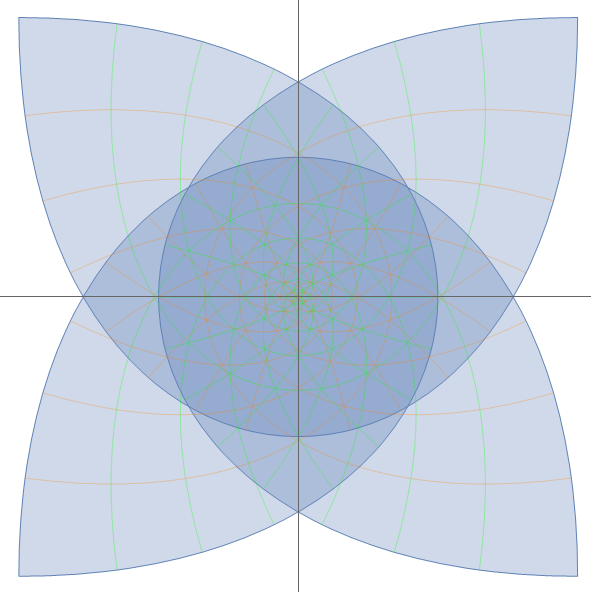# Wolfram Mathematica 对复变函数的另一种可视化方法

``````range=6;
f[x_]:=(x)^3;
ListAnimate[Table[ParametricPlot[{Re[f[u+I*v]*an^2+(u+I*v)*(1-an^2)],Im[f[u+I*v]*an^2+(u+I*v)*(1-an^2)]},{u,-range,range},{v,-range,range},MeshStyle->{Orange,Green},PlotRange->Full,Mesh->Automatic],{an,0,1,1/60}],30]``````

#2   test

## Wolfram Mathematica 混沌摆模拟以及可视化

2022-2-13 20:18:58

## 基于蒙特卡罗方法的一维意见传播模型

2023-1-26 23:46:33

9 条回复 A文章作者 M管理员
1. 牛逼啊

• 其实我一直想做个能互动的项目，你们可以自己输入表达式来观察变化，而不是这样看我的文章。。但是貌似比较难，最近正在想办法搞。。

• 我最近在做一个黎曼zeta函数可视化的项目,想借鉴一下你的方法

• 这里我用两种方式可视化了黎曼\[Zeta]函数，3D图像放在这里
1. (unnamed) – Wolfram Cloud 这个三维图像可以在网页上旋转视角观察的
2.(unnamed) – Wolfram Cloud
我觉得在复杂的函数下使用我这个可视化方法不太有用，主要是变换太复杂了导致不能有效看出变化过程

2. 666

3. 可以可以

4. yysy，mathematica求积分是真的方便（虽然这不是主要功能）本数学系人激推！

• 是这样的，wolfram的符号计算优化做得非常棒，比起matlab和其他软件都要强上不少。

5. 本人也是工科學生，上課時教授拚了命的推薦Matlab，但發了作業我還是用Wolfram解🤣